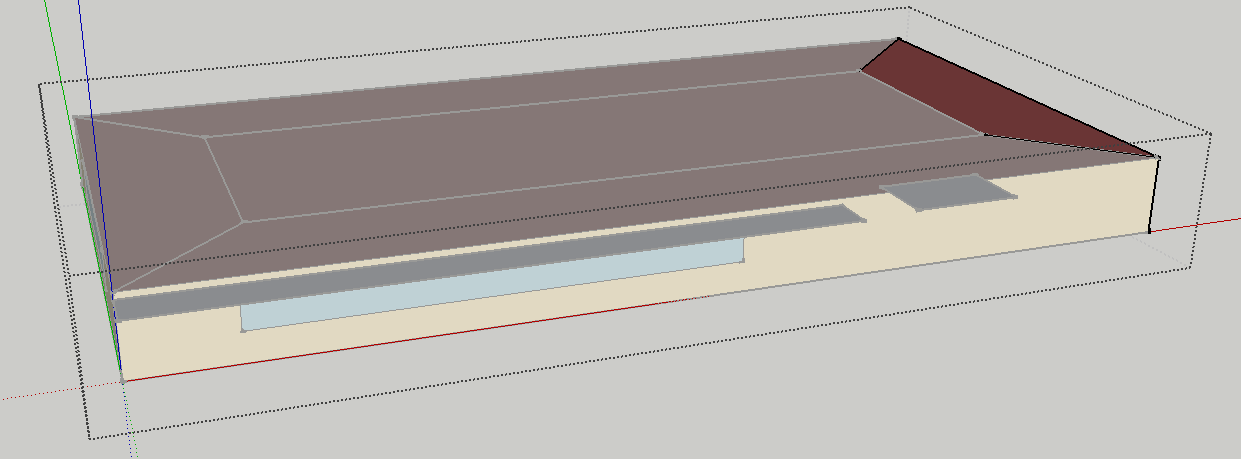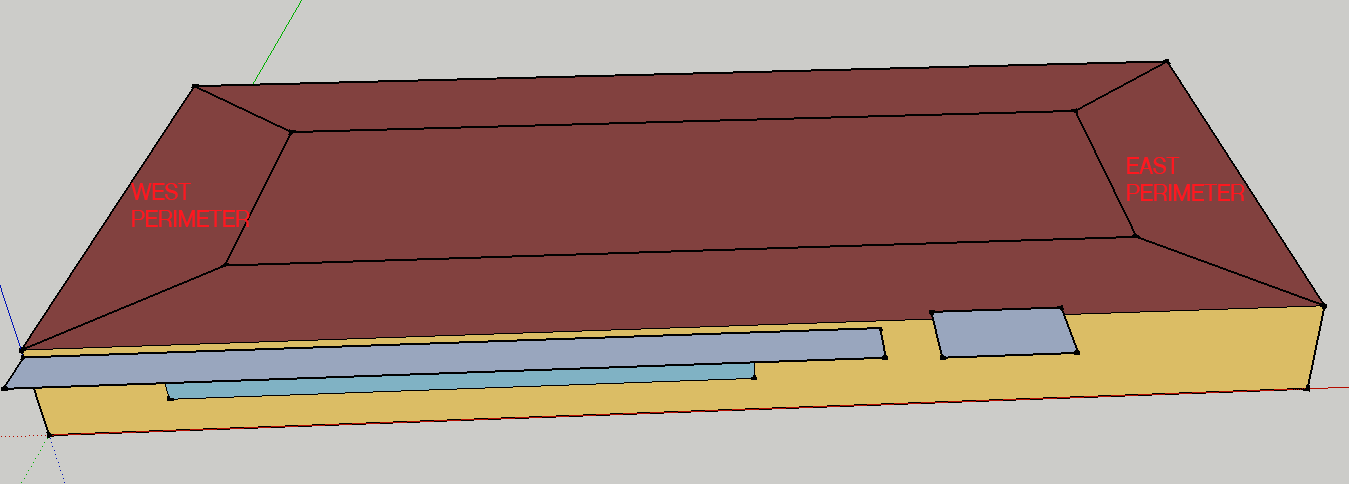Question-and-Answer Resource for the Building Energy Modeling Community
Get s tarted with the Help page

# Differences between relative and world coordinate system?

I have a doubt related to the Coordinate System. Looking at the examples files, for instance, the Exercise2C-Solution.idf (see related doc in Getting Started), used Relative, and the origin of all zones is x = 0, y = 0 and z = 0. I don't understand why, agree with me, in that way the Zone Origin coordinates are not specified relative to the Building Origin.

Using any of them (Relative or World), the vertex of the surfaces continues the same?

edit retag close merge delete

Could you always try to tag your question with a specific software if that's appropriate please? A linked to the example in question is useful. I went ahead and made the changes.

Sort by » oldest newest most voted

The Zones indeed all have the same origin at (0,0,0). It helps to visualize this stuff, here I loaded the model and removed the plenum zone in order to see things better. I've selected the zone that's the furthest away from the building origin (which is located at the intersection of the red, blue and green axis). The zone origin indeed is at (0,0,0).It doesn't matter what the zone origin is as long as the Surfaces are entered properly.

If we look at the difference between the EAST PERIMETER and WEST PERIMETER ZonesHere's the min/max values for each coordinate (x, y, z) for the walls (see code to generate this below)

 (main)> west_walls
=> {:all_x=>[0.0, 3.7], :all_y=>[0.0, 15.2], :all_z=>[0.0, 2.4]}
 (main)> east_walls
=> {:all_x=>[26.8, 30.5], :all_y=>[0.0, 15.2], :all_z=>[0.0, 2.4]}


So the walls for the "EAST PERIMETER" zone have much higher x coordinates than "WEST PERIMETER" walls, which explains why they are placed where they are even though both Zones have the same origin.

### Code to generate the above:

workspace = OpenStudio::Workspace.load('Exercise2C-Solution.idf').get

def get_xyz(w)
all_x = []
all_y = []
all_z = []
for i in 0..w.numExtensibleGroups-1
e = w.getExtensibleGroup(i)
all_x << e.getDouble(0).get
all_y << e.getDouble(1).get
all_z << e.getDouble(2).get
end

return all_x, all_y, all_z
end

west = workspace.getObjectByTypeAndName("Zone".to_IddObjectType, "WEST PERIMETER").get
east = workspace.getObjectByTypeAndName("Zone".to_IddObjectType, "EAST PERIMETER").get

#west_walls = {:walls=>[], :all_x=>[], :all_y=>[], :all_z=>[]}
#east_walls = {:walls=>[], :all_x=>[], :all_y=>[], :all_z=>[]}

west_walls = {:all_x=>[], :all_y=>[], :all_z=>[]}
east_walls = {:all_x=>[], :all_y=>[], :all_z=>[]}

workspace.getObjectsByType("BuildingSurface:Detailed".to_IddObjectType).each do |w|
if w.getString(1).to_s.upcase == 'WALL'
if w.getString(3).to_s.upcase == west.name.to_s.upcase
all_x, all_y, all_z = get_xyz(w)
west_walls[:all_x] += all_x
west_walls[:all_y] += all_y
west_walls[:all_z] += all_z

elsif w.getString(3).to_s.upcase == east.name.to_s.upcase
#east_walls[:walls] << w
all_x, all_y, all_z = get_xyz(w)
east_walls[:all_x] += all_x
east_walls[:all_y] += all_y
east_walls[:all_z] += all_z
end
end
end

west_walls.each {|k, v| west_walls[k] = v.minmax}
east_walls.each {|k, v| east_walls[k] = v.minmax}

more